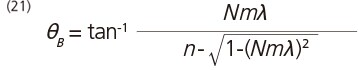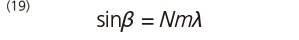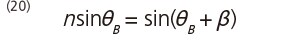Transmission Gratings

In general, transmission gratings are used in the way shown in Fig. 11, with light entering at an angle perpendicular to the reverse side of the grating. In this case, α = 0 and so grating equation (8) gives the following:Fig. 11 Transmission GratingLight is refracted at the facet face of the grooves and by Snell's Law the following equations is given:Here, n is the refractive index of resin and θB is the blaze angle. Combining equations (19) and (20) gives the following:The reflective Littrow-configuration blaze wavelength, λB(Litt), corresponding to the wavelength λ to be picked out with a high efficiency can be obtained by substituting θB into equation (11).
If θB + β ≥ 90°, however, diffracted light cannot be obtained. Also, internal reflection can cause stray light and so this method is not suitable for high-precision spectroscopy.